# Compressibility Options and Buoyancy Forces for Flow Simulations

August 22, 2016

The numerical analysis of fluid flow and temperature fields can offer valuable insight in many engineering applications. Efficiency, of course, is important when performing such simulations. Here, we’ll discuss the various formulations for fluid flow equations in the COMSOL Multiphysics® simulation software and when it’s best to use each option, paying particular attention to how this selection influences heat transfer analyses. We will also cover how to set up both natural and forced convection simulations using these formulations.

### Compressibility Options for the Navier-Stokes Equations in COMSOL Multiphysics®

When it comes to describing the velocity and pressure fields inside the system you are analyzing, there are many equations that could be appropriate. You could, for example, adequately describe a fluid slowly moving in a porous bed with Darcy’s law. But if the fluid moves rapidly, you may need to use the Brinkman equation. While there are many options available, today we will focus on the Navier-Stokes equations, as they are the most common in fluid flow analysis. Note that most of the explanations and practices highlighted here will also apply to the equations referenced above.

The first step is to characterize the type of flow that you are modeling based on fluid density. All fluids are compressible, that is, their density depends on absolute pressure and temperature through a thermodynamic relation, \rho = \rho (pA,T). However, from a practical point of view, most liquids can be safely described as having a density that depends uniquely on temperature, \rho = \rho (T). Density is, of course, a function of an alternate element in some cases — for example, salt concentration in the Elder problem.

In the Single-Phase Flow interface available in COMSOL Multiphysics, there are three possible formulations for momentum and mass conservation equations: Compressible flow (Ma < 0.3), Weakly compressible flow, and Incompressible flow. You can easily select from these compressibility options within the Laminar Flow settings, as highlighted below.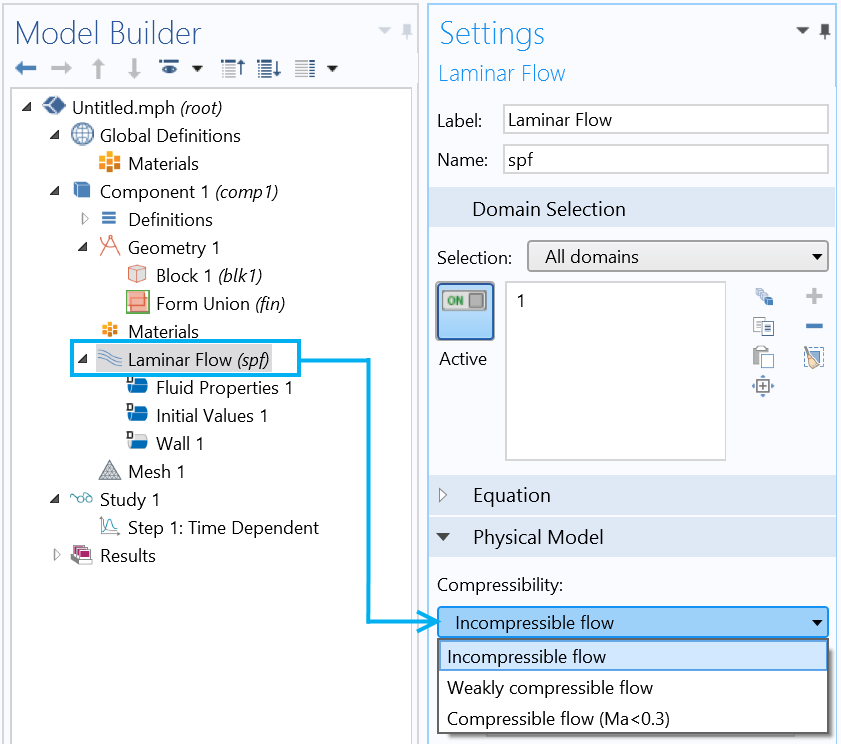Choosing a compressibility option in COMSOL Multiphysics.

In general, the various properties of a fluid are not constant and may depend on a number of quantities. Whether it is necessary to account for such dependencies in your modeling processes is up to you. Since the focus is on mass, momentum, and energy conservation equations in this blog post, we will review how COMSOL Multiphysics deals with viscosity \mu, density \rho, thermal conductivity k, and heat capacity C_p for the different compressibility options.

We’ll begin our discussion with isothermal flow simulations and later turn our attention to nonisothermal cases.

Pumps, mixers, airfoils, and multiphase systems… These are just some of the devices that are often modeled as isothermal. Isothermal flow simulations assume that \mu, \rho, k, and C_p are not dependent on temperature. If the properties are defined as a function of T, they are evaluated at the reference value. This means that the energy equation is weakly coupled with the other two equations through the convective term, and it can be computed at a later time, if needed. It is, however, not always possible to make such an approximation.

Given the freedom that the user interface (UI) of COMSOL Multiphysics grants us, we can first study and solve a single-physics problem and then build a multiphysics problem on top of the initial solution. Keep in mind that neglecting the energy conservation equation, even if you’re not directly interested in the temperature field, is valid only below a certain Mach number (Ma<0.3).

Let’s take a look at how to select the appropriate compressibility option for your modeling case.

#### Choosing the Appropriate Compressibility Option for Isothermal Flows

##### Compressible Flow (Ma < 0.3)

Compressible flow (Ma < 0.3), the most general case, makes no assumptions for the system that is being solved. COMSOL Multiphysics takes into account any dependency that the fluid properties may have on the variables. In isothermal flows, temperature is typically uniform and fluid properties (density and viscosity) remain constant, respectively, evaluated at the reference value. Even so, the properties can still vary with pressure or other quantities, such as concentration. With this formulation, which is the most computationally expensive, we can model any kind of flow and also describe incompressible situations. Our Flow Around an Inclined NACA 0012 Airfoil tutorial model provides one example of how to use the compressible flow formulation.

##### Weakly Compressible Flow

For the Weakly Compressible flow option, new with COMSOL Multiphysics® version 5.2a, the equations look the same as they do for the Compressible flow (Ma < 0.3) option. The only difference is that if the density is pressure dependent, the density will be evaluated at the reference absolute pressure. In this case, all the other dependencies of the density, like species concentration, are accounted for, so we can still use this formulation to account for volume forces given by concentration gradients.

##### Incompressible Flow

The Incompressible flow formulation, meanwhile, is valid whenever \rho can be regarded as constant (i.e., when modeling isothermal liquids or gases at low velocities). This option is also used in immiscible two- or three-phase flow simulations where density is constant. When applying the Incompressible flow formulation, COMSOL Multiphysics automatically uses the reference temperature and pressure to evaluate \rho. In addition, it uses the reference temperature to evaluate \mu. Of course, there are many applications in which the quantities mentioned above are dependent on another variable, like a species concentration. In these cases, the density must be explicitly evaluated at a reference value for these variables within the interface. To learn more, download our Water Purification Reactor model example.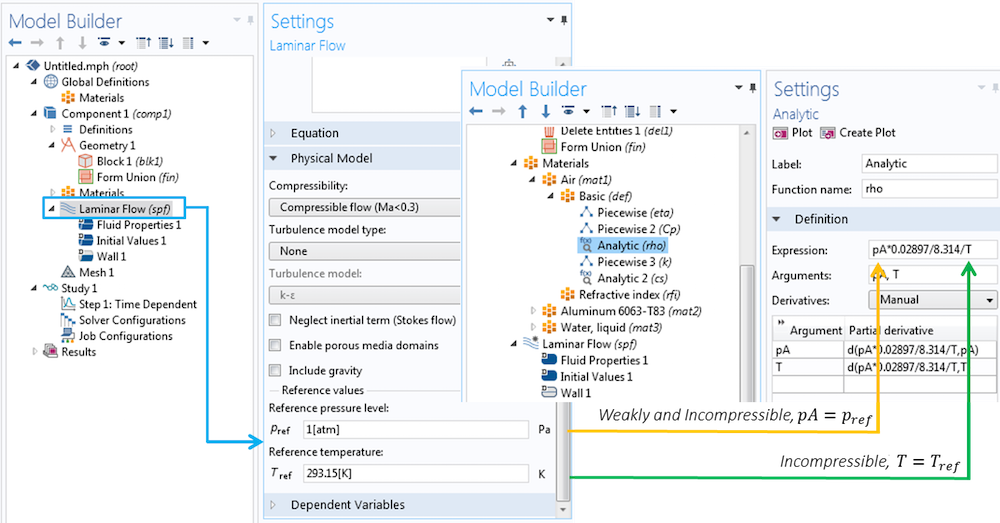Density specification in the case of isothermal weakly compressible and incompressible flows.

Also note that the form of the equations, which are shown within the Equation section, change according to the selected option.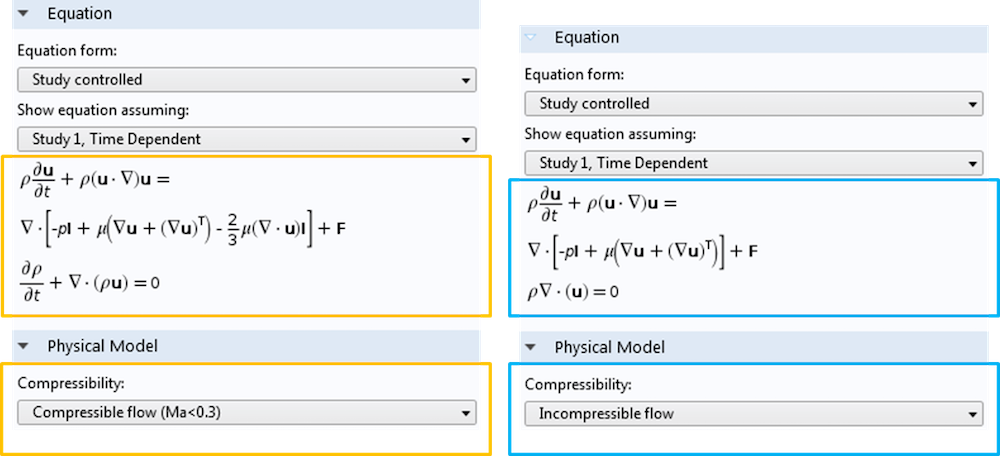The different formulations for compressible and incompressible Navier-Stokes equations.

Nonisothermal flow simulations typically relate to cooling and heating applications, namely conjugate heat transfer. These simulations can refer to systems that are governed by natural, forced, or mixed convection.

Depending on the type of system that is being analyzed and the hypothesis that is assumed to be true, any of the compressible options can be appropriate for nonisothermal simulations. Since Compressible flow (Ma < 0.3) is the only meaningful formulation for gases subject to high pressure changes, we will focus here on systems that are below the Mach number limit, and those with fluid properties that are uniquely dependent on temperature. (There is a dedicated interface available for modeling high Mach number systems, as highlighted in this Sajben diffuser tutorial model.) The system of equations — mass, momentum, and energy conservation — is completely coupled, as the velocity appears inside the energy equation. Meanwhile, the pressure will appear explicitly in the momentum equation; the temperature will appear explicitly in the energy equation; and both temperature and pressure may be inside the fluid properties in these two equations.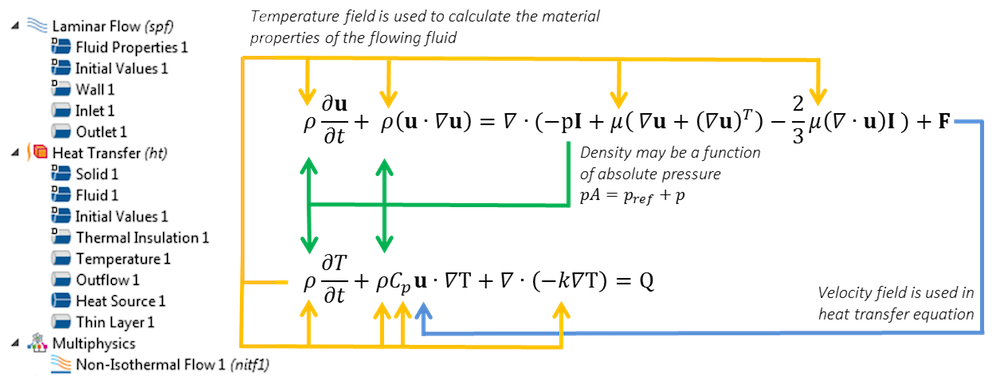Couplings of momentum and energy equations. In the case of natural convection, a part of the volume force F depends on temperature gradients.

#### Choosing the Appropriate Compressibility Option for Nonisothermal Flow Simulations

##### Compressible Flow (Ma < 0.3)

For convective heat transfer simulations, the Compressible flow (Ma < 0.3) option can be used to analyze forced and natural convection.

Forced convection refers to when the properties of the fluid vary in a nonnegligible way from pressure and temperature. This is the case for high-speed systems where the pressure changes are nonnegligible in their influence on density. As previously noted, the density of liquids rarely depends on pressure, which makes this exactly the same as the Weakly compressible flow formulation. See our Shell-and-Tube Heat Exchanger tutorial model to learn more.

In natural convection, the driving force is the buoyancy force due to temperature gradients. The Compressible flow (Ma < 0.3) option must be used for gases in closed cavities in order for the system of equations to be consistent. In fact, if the volume cavity and total mass are constant, then the average density needs to be constant. Pressure changes help balance out density variations that are caused by temperature variations. Interested in modeling such a system? Refer to our Free Convection in a Light Bulb tutorial, which exemplifies the set up of transient conjugate heat transfer models with radiation.

##### Weakly Compressible Flow

The Weakly compressible flow option comes with a simplified formulation that usually leads to increased computational speed. It is the default option when the predefined Nonisothermal Flow or Conjugate Heat Transfer coupling is opened in COMSOL Multiphysics. This formulation, which basically neglects the green arrow coupling shown in the previous image, can be used to analyze forced and natural convection.

In the case of forced convection, the Weakly compressible flow option can be applied to the simulation of water or other fluids and is often valid for modeling gases in open systems (see this heat sink model example). The same considerations are valid for natural convection, as demonstrated in our vacuum flask tutorial.

##### Incompressible Flow

The Incompressible flow option can be applied to both forced and natural convection as well. The initial case applies when you want to make simulations in cascade. For instance, sometimes it is interesting to compute the flow field at a reference temperature and then compute the temperature field in a second simulation. This can provide a powerful approximation when fluid properties do not vary much within the simulation’s temperature and pressure range. One good candidate for this type of modeling is a heat exchanger that includes liquids. You can also apply this approach to obtain more consistent initial values for highly nonlinear stationary problems. After computing the flow field and temperature field with ‘frozen velocity’, it is possible to gain considerable convergence improvements by using the initial values for the fully coupled simulations.

For the former case, COMSOL Multiphysics implements the Boussinesq approximation. The reference temperature and pressure, specified in the interface, are used to compute density, viscosity, heat capacity, and thermal conductivity. Further, the software automatically computes the coefficient of thermal expansion for the fluid, \alpha_p, taking the derivative of the density around the reference temperature, T_{ref}, and uses it to impose the buoyancy force, F_b=-\rho_{ref}\alpha_p(T_{ref})(T-T_{ref})\textbf{g}, where \textbf{g} denotes the gravity vector. You also have the option to enter the coefficient directly, as shown below.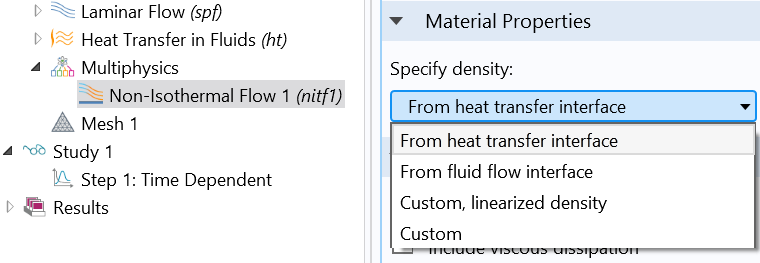Options for specifying the density.

#### The Importance of Boundary Conditions

When utilizing the gravity option, an important concern is the need for consistent boundary conditions and initial values, particularly when dealing with natural convection simulations. This could be a nontrivial task since a domain force, the buoyancy force, is working inside the system, and we need to account for its presence. Consider, for instance, a system like a pipe where a hydrostatic head is present. Here, it is clear that we simply can’t impose a constant pressure as a boundary condition if the boundary itself is not perpendicular to the gravity vector.

Besides enabling you to model all of the above systems and situations, COMSOL Multiphysics helps to address initial values and boundary conditions for each case. To learn more, check out our Gravity and Boundary Conditions tutorial model.

### How to Model Natural and Forced Convection in COMSOL Multiphysics®

For forced convection, the flow and temperature coupling is taken care of at the Multiphysics node level. Within the Non-Isothermal Flow interface, the equations are coupled and fluid flow and heat transfer properties are synchronized (see the screenshot below). Depending on the compressibility option that is chosen, COMSOL Multiphysics will operate in the background to make the appropriate changes for the fluid properties, making them consistent with the selected formulation. Additionally, the Non-Isothermal Flow interface takes care of implementing thermal wall functions and computing turbulent heat conduction.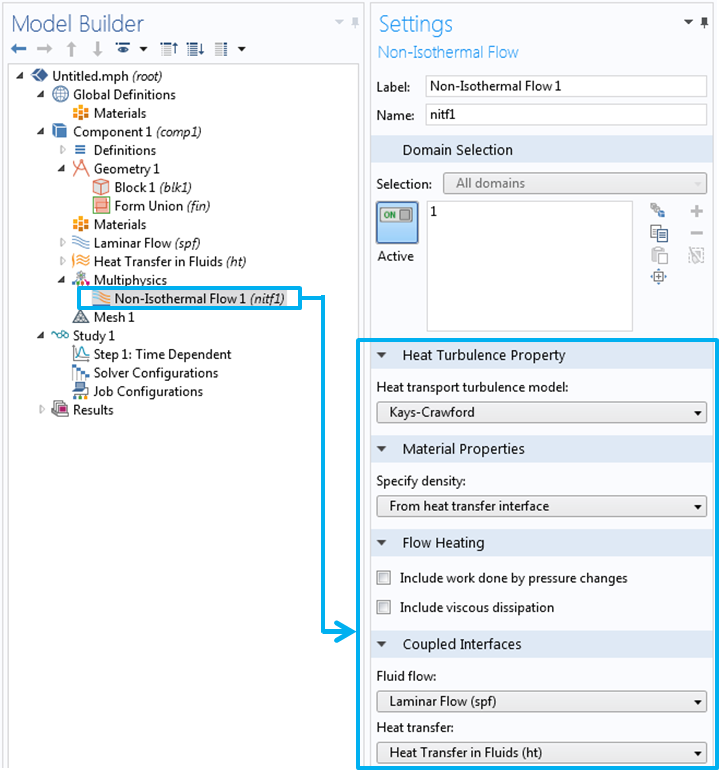Settings window for the Non-Isothermal Flow interface.

If it is necessary to include a buoyancy force due to temperature or concentration gradients, then you must select the Include gravity check box. This will generate values in the Reference Values section that can be used to compute the hydrostatic pressure approximate, alongside reference temperature and pressure. Selecting the Include gravity check box also causes a new subnode to appear: Gravity. Here, you can specify the direction of the acceleration acting on the system. When the Gravity subnode is added, the hydrostatic contribution taken at the reference temperature and pressure is automatically added inside the boundary conditions, if appropriate.

To model natural convection, it is simply necessary to use both the Gravity feature and Non-Isothermal Flow interface. Together, they model flow and temperature fields coupled in the presence of gravity acceleration.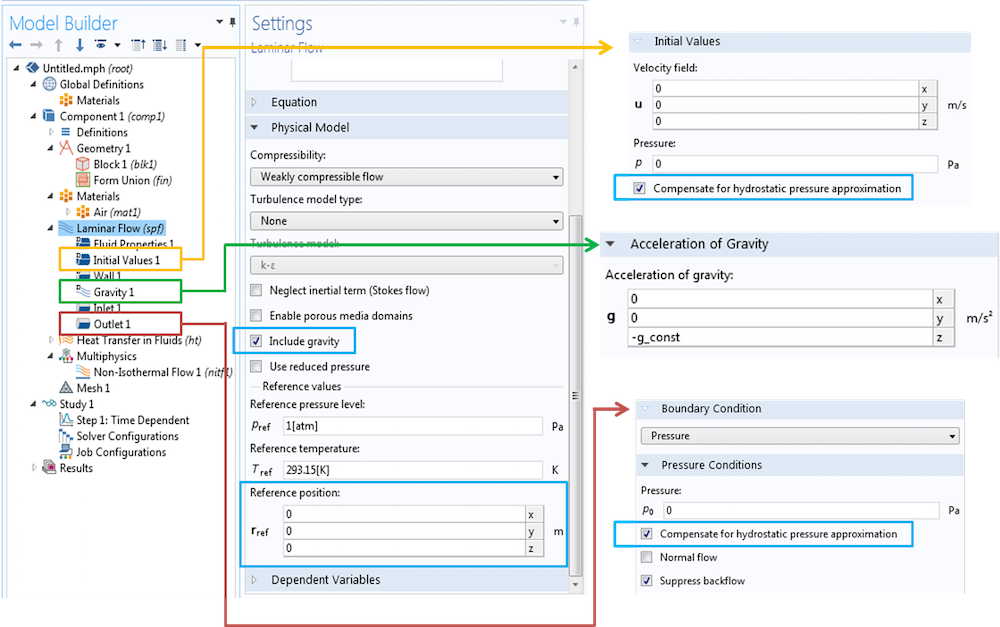Changes prompted by selecting the Include gravity check box.

The following simulation plots correlate with our vacuum flask tutorial model, which evaluates the thermal performance of a bottle holding hot fluid. This system is composed of a gas, air, outside the flask that is flowing in an open system — the flask is leaning on a table in a wide room. Such considerations make this example a helpful resource for understanding the use, assumptions, and results of the various formulations. The Compressible flow (Ma < 0.3) option, for instance, is always applicable. Since air is flowing in an open system, the Weakly compressible flow option is also applicable. And lastly, because the density changes are small, the Incompressible flow option can represent the system appropriately as well.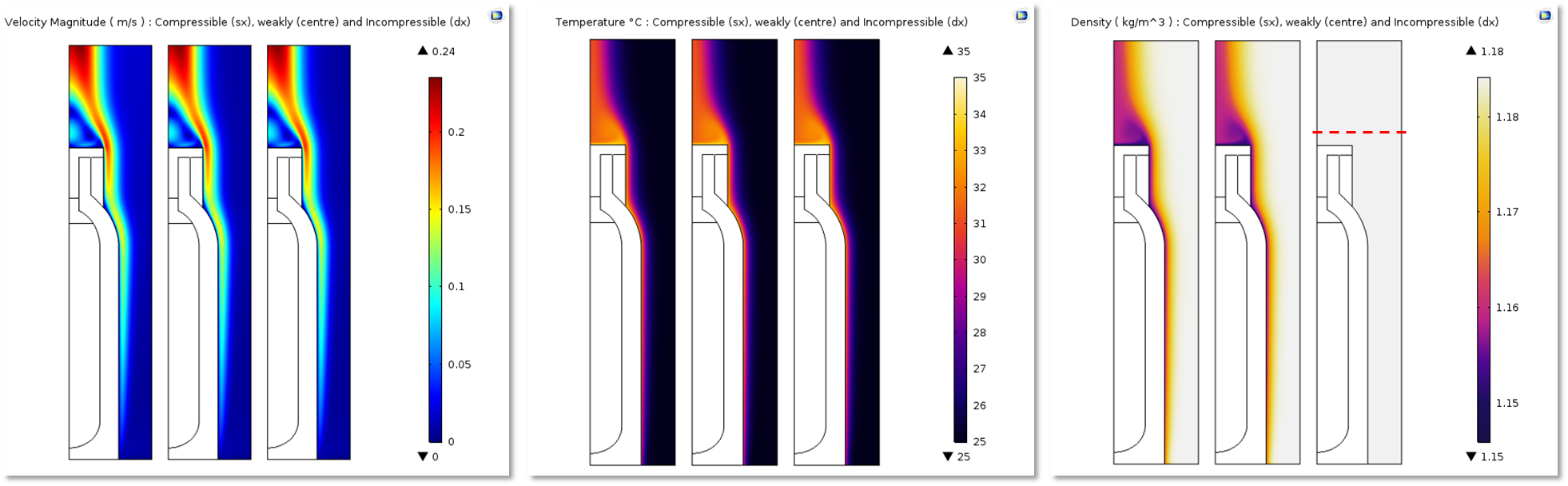Plots comparing velocity, temperature, and density fields (respectively) for simulations using the Compressible flow (Ma < 0.3), Weakly compressible flow, and Incompressible flow formulations. We performed these simulations by simply toggling between the three compressibility options.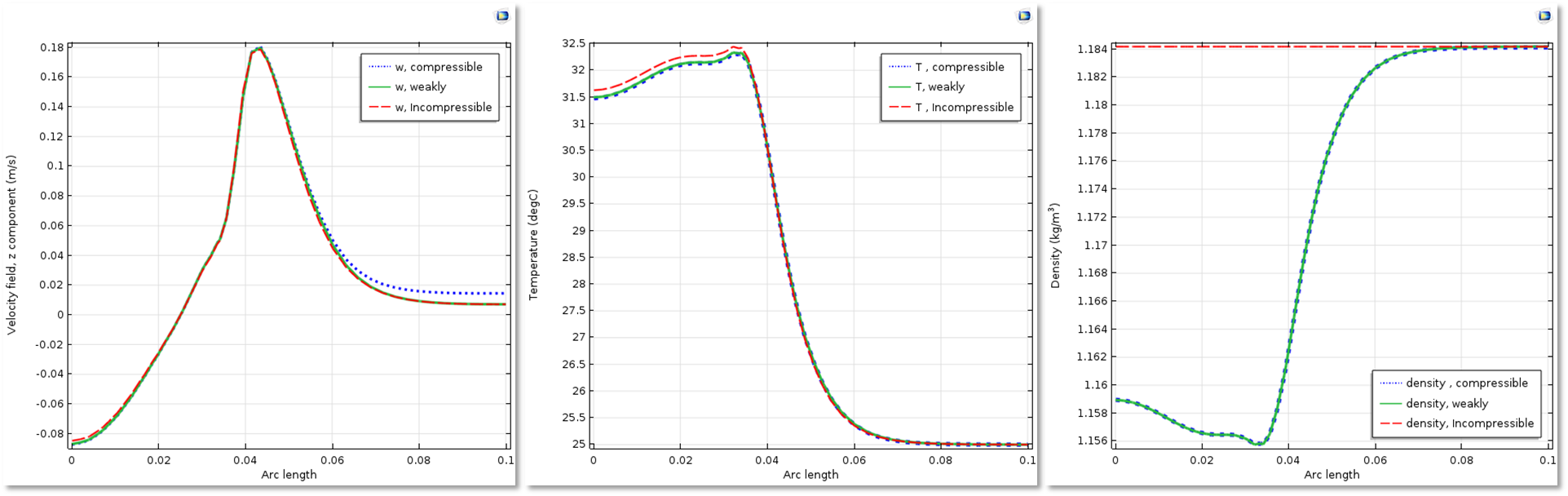Graphs comparing velocity, temperature, and density fields (respectively) for simulations using the Compressible flow (Ma < 0.3), Weakly compressible flow, and Incompressible flow formulations. We performed these evaluations at the red dashed line, shown in the plot on the right in the previous set of images, after a simulation time of 10 hours.

### Concluding Remarks on Selecting Compressibility Options

Choosing the correct compressibility option is key for solving your system in an accurate and efficient way. COMSOL Multiphysics provides you with functionality that allows you to model both natural and forced convection with ease, while still offering various modeling choices and giving you complete control over the simulations at hand. This results in an optimized approach to the numerical analysis of fluid flow and temperature fields, further advancing your engineering design.

Use the table below as a helpful guide for choosing the compressibility option that is most appropriate for your modeling needs.

Compressibility Option Isothermal Flow Nonisothermal Flow
Compressible flow (Ma < 0.3)
• Nonnegligible density changes due to pressure
• Air, ideal gas
• Nonnegligible density changes due to pressure
• Air, ideal gas
• Gas in closed cavities
Weakly compressible flow
• Account for density dependence on any variable (neglecting pressure dependency)
• Often valid for gas or air flowing in open systems
• Water, liquids
Incompressible flow
• Constant density
• Boussinesq approximation

### Further Resources on Modeling Fluid Flow and Heat Transfer in COMSOL Multiphysics®

#### Categories##### Pei Yi Soh
March 7, 2018

Hi,
Is the “include gravity” option available in Comsol v5.2??
Ty!##### Beatrice Carasi
March 13, 2018

Hi Pei,

the gravity option was introduced in version 5.2a, the one that was released after the one you mentioned. If you have the possibility to update, I strongly recommend you to do so since it is much easier from 5.2a to model natural convection. All the setting update automatically accordingly to the choice to activate the gravity checkbox (material definition, solver choice…). Anyway the most important feature is the automatic definition of the boundary condition containing the hydrostatic part.

Happy Modeling ,

Beatrice,
COMSOL##### Youssef Aider
February 13, 2019

thank youBeatrice Carasi for the explanation,
i just want to ask about the case where the density of the fluid is defined as a function temperature. for example argon gas for the plasma modeling, which has a predefined density in the material properties as function of the temperature, if we use compressible flow, will that interfere with the predefined material properties? and give the inconsistent initial condition error?
thanks##### Beatrice Carasi
February 14, 2019

Hi Youssef,

the blog covers in almost its entirety a density that depends on temperature inside the material definition. The compressibity options only define how this dependence is read: incompressible sets a constant density, weakly compressible only retains the temperature dependence and compressible allows the density to depend on both temperature and pressure.
Plasma modeling contains a lot of complexity and it’s a challenging simulation 🙂 .
If you have an error that reads fail to find consistent initial values for a transient simulation, the most likely reason is that the dirichelet boundary conditions do not match the initial values inside the domain for some equation you are solving. If you need assistence with a model I’d suggest you to write to support@cosmol.com, in case you are using a license that is under subscription.

Happy modeling,

Bea##### Youssef Aider
May 8, 2019

Beatrice,
thank you for the reply. I finally made it to work. yes the inconsistent initial conditions were related to weak boundary conditions.##### Atabak Pourreza
March 6, 2020

Hi,
I am modeling a simple rectangular flow duct with SST model, I could solve it for incompressible and weakly compressible flow without any issue but I need to move to compressible flow (Ma<0.3). when I solve it I encounter an error message as below:

Turbulence variables
Attempt to evaluate the square root of a negative argument.
– Function: sqrt
Error in evaluating the variable.
– Variable: comp1.spf.uTauVisc
– Defined as: sqrt ((sqrt ((((comp1.spf.u_tangx ^ 2) + (comp1.spf.u_tangy ^ 2)) + (comp1.spf.u_tangz ^ 2)) + eps) * down (comp1. spf.nu)) / comp1.spf.delta_w)
Error in evaluating the variable.
– Variable: comp1.spf.uStar
– Defined as: sqrt (sqrt ((comp1.spf.uTauVisc ^ 4) + (comp1.spf.uStarLog ^ 4))))
Error in evaluating the variable.
– Variable: comp1.spf.omLog
– Defined as: comp1.spf.uStar / (comp1.spf.delta_w * (comp1.spf.kappav * sqrt (comp1.spf.beta0s))))
Error in evaluating the variable.
– Variable: comp1.spf.om_w
– Defined as: sqrt ((comp1.spf.omVisc ^ 2) + (comp1.spf.omLog ^ 2))
Error in evaluating expression.
– Expression: -comp1.om + comp1.spf.om_w

the highest speed of the simulation is below Mach 0.3.

Thanks,##### Beatrice Carasi
March 6, 2020 COMSOL Employee

Hi Atabak,

this is a question more appropriate for COMSOL support. Please do write to support@comsol.com .

Happy modelling,
Beatrice – COMSOL

EXPLORE COMSOL BLOG